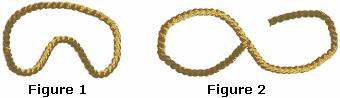## Definition Of Curve

Curve is a line that is not straight.

A curve can be classified into two categories, an open curve or a closed curve.

### Example of CurveFigure 1 is a closed curve and Figure 2 is an open curve.

### Solved Example on Curve

#### Ques: Which of the following is an open curve?

##### Choices:

A. circle
B. ellipse
C. parabola
D. none of the above